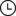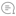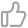# 深度学习中的 Attention 机制总结与代码实现（2017-2021年）极市平台 |4412021-06-13 09:03000
↑ 点击蓝字 关注极市平台## 项目地址

https://github.com/xmu-xiaoma666/External-Attention-pytorch
（点击阅读原文即可跳转）

## 1. External Attention

### 1.1. 引用

Beyond Self-attention: External Attention using Two Linear Layers for Visual Tasks.---arXiv 2021.05.05

### 1.4. 使用方法

``from attention.ExternalAttention import ExternalAttentionimport torchinput=torch.randn(50,49,512)ea = ExternalAttention(d_model=512,S=8)output=ea(input)print(output.shape)``

## 2. Self Attention

### 2.1. 引用

Attention Is All You Need---NeurIPS2017

### 2.4. 使用方法

``from attention.SelfAttention import ScaledDotProductAttentionimport torchinput=torch.randn(50,49,512)sa = ScaledDotProductAttention(d_model=512, d_k=512, d_v=512, h=8)output=sa(input,input,input)print(output.shape)``

## 3. Squeeze-and-Excitation(SE) Attention

### 3.1. 引用

Squeeze-and-Excitation Networks---CVPR2018

### 3.4. 使用方法

``from attention.SEAttention import SEAttentionimport torchinput=torch.randn(50,512,7,7)se = SEAttention(channel=512,reduction=8)output=se(input)print(output.shape)``

## 4. Selective Kernel(SK) Attention

### 4.1. 引用

Selective Kernel Networks---CVPR2019

### 4.4. 使用方法

``from attention.SKAttention import SKAttentionimport torchinput=torch.randn(50,512,7,7)se = SKAttention(channel=512,reduction=8)output=se(input)print(output.shape)``

## 5. CBAM Attention

### 5.1. 引用

CBAM: Convolutional Block Attention Module---ECCV2018

### 5.3. 简介

Channel Attention方面，大致结构还是和SE相似，不过作者提出AvgPool和MaxPool有不同的表示效果，所以作者对原来的特征在Spatial维度分别进行了AvgPool和MaxPool，然后用SE的结构提取channel attention，注意这里是参数共享的，然后将两个特征相加后做归一化，就得到了注意力矩阵。

Spatial Attention和Channel Attention类似，先在channel维度进行两种pool后，将两个特征进行拼接，然后用7x7的卷积来提取Spatial Attention（之所以用7x7是因为提取的是空间注意力，所以用的卷积核必须足够大）。然后做一次归一化，就得到了空间的注意力矩阵。

### 5.4. 使用方法

``from attention.CBAM import CBAMBlockimport torchinput=torch.randn(50,512,7,7)kernel_size=input.shapecbam = CBAMBlock(channel=512,reduction=16,kernel_size=kernel_size)output=cbam(input)print(output.shape)``

## 6. BAM Attention

### 6.1. 引用

BAM: Bottleneck Attention Module---BMCV2018

### 6.3. 简介

Channel Attention方面，与SE的结构基本一样。Spatial Attention方面，还是在通道维度进行pool，然后用了两次3x3的空洞卷积，最后将用一次1x1的卷积得到Spatial Attention的矩阵。

### 6.4.使用方法

``from attention.BAM import BAMBlockimport torchinput=torch.randn(50,512,7,7)bam = BAMBlock(channel=512,reduction=16,dia_val=2)output=bam(input)print(output.shape)``

## 7. ECA Attention

### 7.1. 引用

ECA-Net: Efficient Channel Attention for Deep Convolutional Neural Networks---CVPR2020

### 7.4. 使用方法：

``from attention.ECAAttention import ECAAttentionimport torchinput=torch.randn(50,512,7,7)eca = ECAAttention(kernel_size=3)output=eca(input)print(output.shape)``

## 8. DANet Attention

### 8.1. 引用

Dual Attention Network for Scene Segmentation---CVPR2019

### 8.4. 使用方法

``from attention.DANet import DAModuleimport torchinput=torch.randn(50,512,7,7)danet=DAModule(d_model=512,kernel_size=3,H=7,W=7)print(danet(input).shape)``

## 9. Pyramid Split Attention(PSA)

### 9.1. 引用

EPSANet: An Efficient Pyramid Split Attention Block on Convolutional Neural Network---arXiv 2021.05.30

### 9.4. 使用方法

``from attention.PSA import PSAimport torchinput=torch.randn(50,512,7,7)psa = PSA(channel=512,reduction=8)output=psa(input)print(output.shape)``

### 10.1. 引用

ResT: An Efficient Transformer for Visual Recognition---arXiv 2021.05.28

### 10.4. 使用方法

``from attention.EMSA import EMSAimport torchfrom torch import nnfrom torch.nn import functional as Finput=torch.randn(50,64,512)emsa = EMSA(d_model=512, d_k=512, d_v=512, h=8,H=8,W=8,ratio=2,apply_transform=True)output=emsa(input,input,input)print(output.shape)``

## 写在最后

△点击卡片关注极市平台，获取最新CV干货

YOLO教程：一文读懂YOLO V5 与 YOLO V4大盘点｜YOLO 系目标检测算法总览全面解析YOLO V4网络结构1.作者保证投稿作品为自己的原创作品。
2.极市平台尊重原作者署名权，并支付相应稿费。文章发布后，版权仍属于原作者。
3.原作者可以将文章发在其他平台的个人账号，但需要在文章顶部标明首发于极市平台△长按添加极市平台小编00

暂无评论~~
Ctrl+Enter